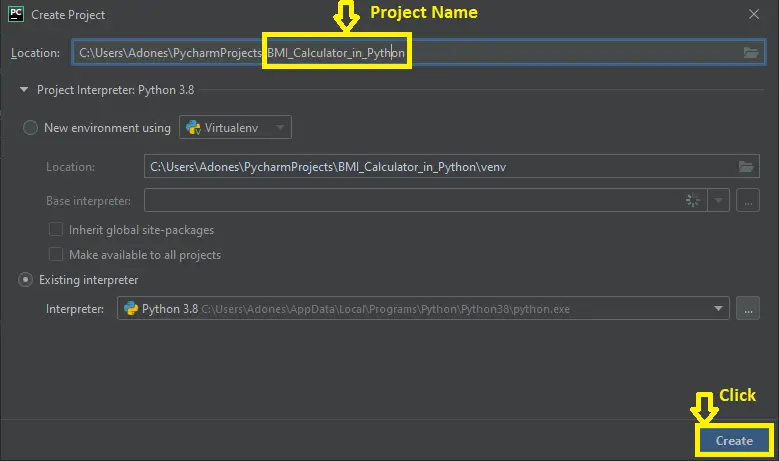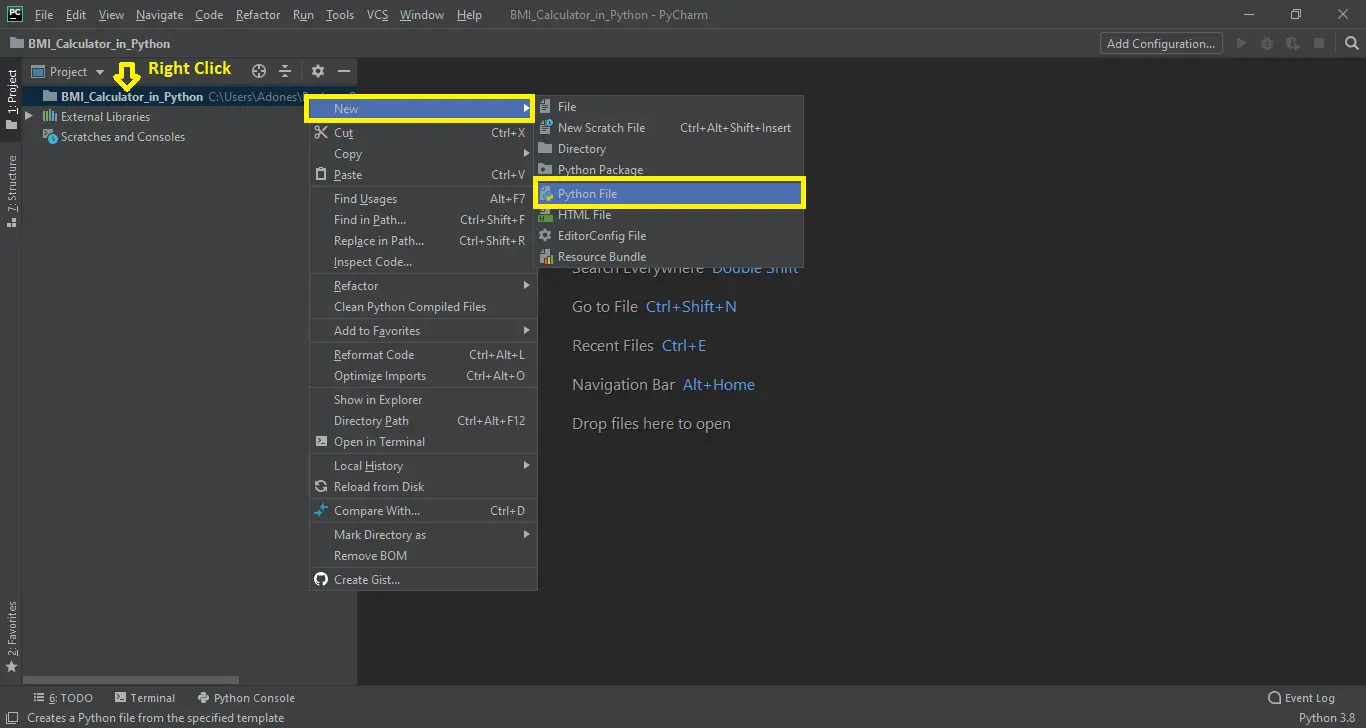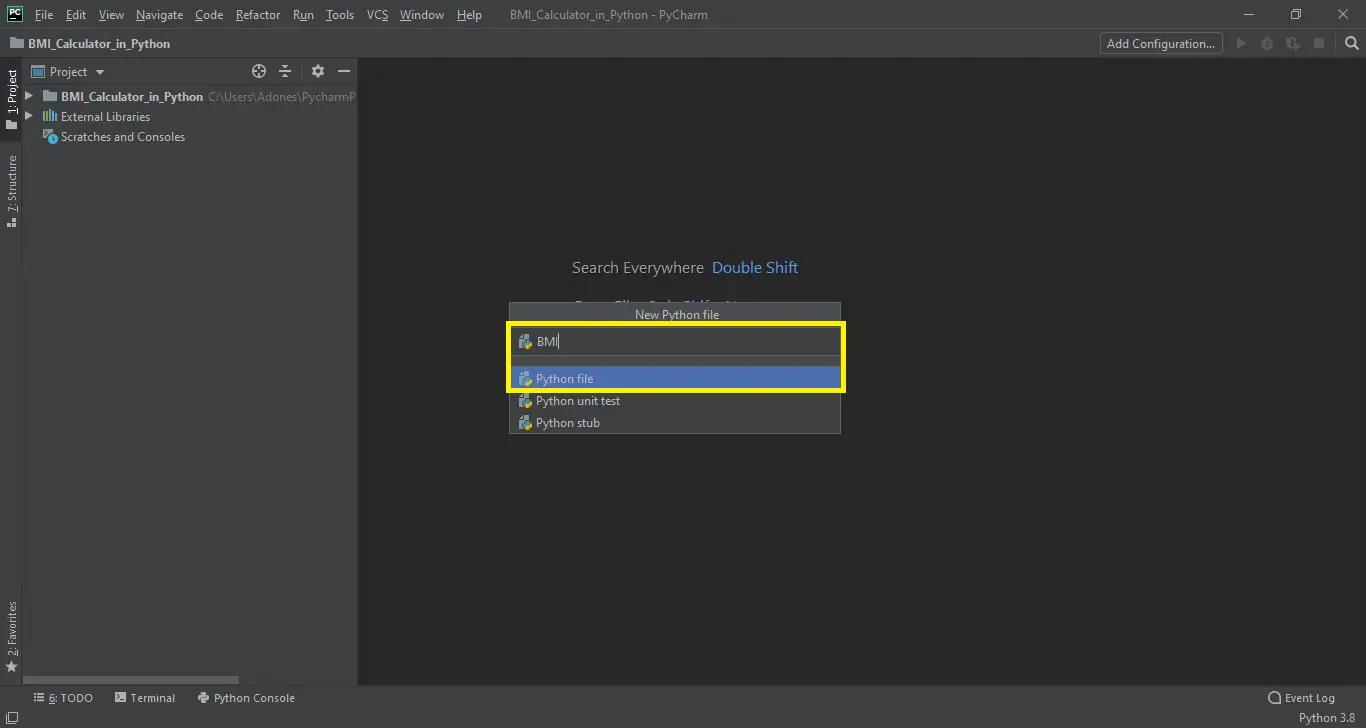BMI Calculator Python Program with Source Code | 2022 - FREE

# BMI Calculator Python Program with Source Code

This BMI Calculator Python is created using tkinter and graphical user interface. The BMI Calculator in Python is free to download the open source code.

The task is for the client advantageous, for measure your body fat or body mass index (BMI). With the BMI esteem, you can check whether you have a solid weight or not. The undertaking record contains a python content (BMI.py).

## BMI Calculator Program in Python – Project Information’s

Discussing the highlights of this Python BMI Calculator framework, this python application is intended to figure the BMI weight, height estimation of client by entering the estimation of their height and weight.

You can simply need to choose the weight and enter the stature you need to include in estimations and snap the catch for the outcome. It is fit for dealing with a wide range of exemptions.

Before you start creating this  BMI Calculator Python, make sure that you have PyCharm IDE installed in your computer.

## Steps on How To Create a BMI Calculator Program in Python with Source Code

Time needed: 5 minutes.

These are the steps on How To Create a BMI Calculator in Python with Source Code

1. Step 1: Create a project name.

First when you finished installed the Pycharm IDE in your computer, open it and then create a “project name” after creating a project name click the “create” button.2. Step 2: Create a python file.

Second after creating a project name, “right click” your project name and then click “new” after that click the “python file“.3. Step 3: Name your python file.

Third after creating a python file, Name your python file after that click “enter“.4. Step 4: The actual code.

This is the actual coding on how to create BMI Calculator in Python, and you are free to copy this code and download the full source code given below.

## Code Explanations

### 1. Importing Tkinter Module

Code:

```from tkinter import *
```

Explanation:

In the code given below, which is for the function for importing tkinter. Tkinter is the standard GUI library for Python. Python when combined with Tkinter provides a fast and easy way to create GUI applications.

### 2. This module is for the BMI calculator

Code:

```def BodyMassIndex_Calculator():
BMI_Height = float(variable2.get())
BMI_Weight = float(variable1.get())
BodyMassIndex = str('%.2f' % (BMI_Weight / (BMI_Height * BMI_Height)))
lbl_res_bmi.config(text=BodyMassIndex)```

Explanation:

In the code given below, which is for the function for BMI calculator.

### 3. This module is for the design for main window

Code:

```Tops = Frame(application, width=1350, height=50, bd=8, relief="raise")
Tops.pack(side=TOP)

frame1 = Frame(application, width=600, height=600, bd=8, relief="raise")
frame1.pack(side=LEFT)

frame2 = Frame(application, width=300, height=700, bd=8, relief="raise")
frame2.pack(side=RIGHT)

frame1A = Frame(frame1, width=600, height=200, bd=20, relief="raise")
frame1A.pack(side=TOP)
frame1B = Frame(frame1, width=600, height=600, bd=20, relief='raise')
frame1B.pack(side=TOP)

lbl_T1 = Label(Tops, text=" BODY MASS INDEX ", padx=16, pady=16, bd=16, fg='#000000',
font=("arial", 54, 'bold'), bg="navajo white", relief='raise', width=32, height=1)
lbl_T1.pack()

lbl_w = Label(frame1A, text="Select Weight in Kilograms", font=('arial', 20, 'bold'), bd=20).grid(row=0, column=0)
b_w = Scale(frame1A, variable=variable1, from_=1, to=500, length=880, tickinterval=30, orient=HORIZONTAL)
b_w.grid(row=1, column=0)

lbl_h = Label(frame1B, text="Enter Height in Meters Square", font=('arial', 20, 'bold'), bd=20).grid(row=0, column=0)
t_h = Entry(frame1B, textvariable=variable2, font=('arial', 16, 'bold'), bd=16, width=22, justify='center')
t_h.grid(row=1, column=0)

lbl_res_bmi = Label(frame1B, padx=16, pady=16, bd=16, fg='#000000', font=('arial', 30, 'bold'), bg='navajo white',
relief='sunk', width=34, height=1)
lbl_res_bmi.grid(row=2, column=0)```

Explanation:

In the code given below, which is for the function for the design of BMI screen windows. You can see the frame, and the label use in the system.

### 4. This module is the design for BMI table

Code:

```lbl_table_bmi = Label(frame2, font=("arial", 20, 'bold'), text='BMI Table').grid(row=0, column=0)
bmi_tab_text_lbl = Text(frame2, height=12, width=38, bd=16, font=("arial", 12, 'bold'))
bmi_tab_text_lbl.grid(row=1, column=0)

bmi_tab_text_lbl.insert(END, 'Meaning \t\t' + "BMI \n\n")
bmi_tab_text_lbl.insert(END, 'Normal weight \t\t' + "19-24 \n\n")
bmi_tab_text_lbl.insert(END, 'Overwight \t\t' + "25-29,9 \n\n")
bmi_tab_text_lbl.insert(END, 'Obesity level I \t\t' + "30-34, 9 \n\n")
bmi_tab_text_lbl.insert(END, 'Obesity level II \t\t' + "35-39, 9\n\n")
bmi_tab_text_lbl.insert(END, 'Obesity level III \t\t' + ">= 40\n\n")```

Explanation:

In the code given below, which is for the function for the design of BMI table.